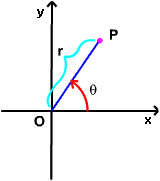A# polar coordinates

Polar coordinates are a coordinate system in which distances are measured from a fixed reference point (the pole), and angles from a fixed reference line. There are two polar coordinations, r and θ, of a point P in the plane, where r is the point's distance from the origin and θ is an angle measured anticlockwise from some reference line emanating from the origin. Spherical polar coordinates incorporate a second angle, φ, which represents the angle of inclination of a line drawn to the point from a plane surface.

A polar equation is one that uses polar coordinates.